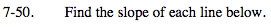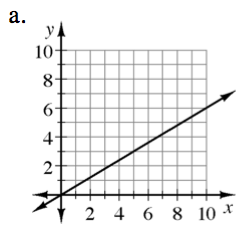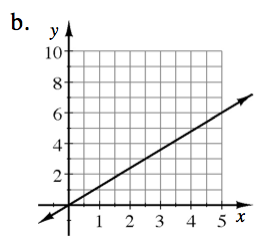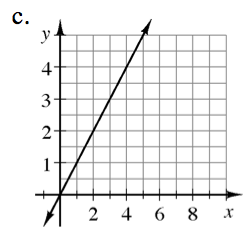### Home > MC2 > Chapter Ch7 > Lesson 7.1.5 > Problem7-50

7-50.Slope can be defined as the change in y over the change in x.

Pick two whole-number points on the line.

The coordinates for these two points are (10, 6) and
(5, 3). Now count the change in y and the change in x.

$\frac{3}{5}$

Pick two points on the line.

These coordinates are (5, 6) and (0, 0).
Find the slope. See part (a) for help.See part (a).